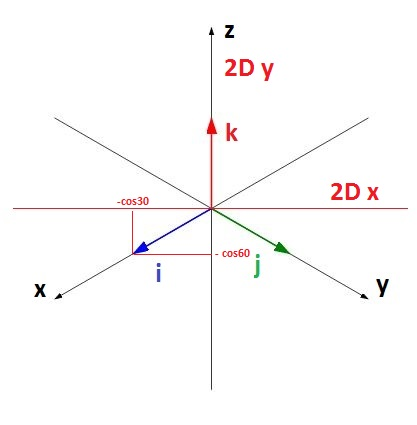# Isometric projection of a vector

This online calculator draws isometric projection of a vector, giving its coordinates in 3D space.

When I've started to write a calculator Cross product calculator, I've planned to visualize the results of cross product on the graph, so that calculator can stand out from the crowd to say so. Thus, I needed to find a way to display a 3D vector on a 2D picture. Surely, I wanted to use isometric projection.

Isometric projection is a method for visually representing three-dimensional objects in two dimensions in technical and engineering drawings. It is an axonometric projection in which the three coordinate axes appear equally foreshortened, and the angle between any two of them is 120 degrees.1

How to draw a vector with three coordinates in 3D space as a vector with two coordinates on a 2D plane? We need to find a formula which converts 3 coordinates x, y, z into 2 coordinates x, y. It turned out to be quite simple. Since each vector in 3D space can be defined as a linear combination of three standard basis vectors, we need to find a formula for converting them and then use 3D vector coordinates as scalar multipliers.

Now let's look at the picture below, which shows how the three standard basis vectors look like in isometric projection (we use a right-handed coordinate system, where the x-axis points to the left, y-axis points to the right)We know that vector i in three dimensional coordinate system has coordinates . If we look at the picture, we can see that its coordinates in two dimensional coordinate system is .
For vector j with it is , and for vector k it is simply .

Now we just need to form a conversion matrix which turns our 3D basis vectors to new 2D vectors when used. And here is the final conversion expression in matrix form:

If we use this matrix to convert basis vectors, we indeed end up with 2d vectors listed above. This conversion matrix is used in the calculator below to display a 3D vector on a 2D picture using isometric projection.#### Isometric projection of a vector

URL copiado para a área de transferência